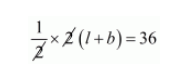# Half the perimeter of a garden, whose length is 4 more than its width is 36 m.`
Question:

Half the perimeter of a garden, whose length is 4 more than its width is 36 m. Find the dimension of the garden.

Solution:

Let perimeter of rectangular garden will be $2(l+b)$.if half the perimeter of a garden will be $36 \mathrm{~m}$$(l+b)=36 \cdots(i)$

When the length is four more than its width then $(b+4)$

Substituting $l=b+4$ in equation $(i)$ we get

$l+b=36$

$b+4+b=36$

$2 b=36-4$

$2 b=32$

$b=\frac{32}{2}$

$b=16$

Putting $b=16$ in equation $(i)$ we get

$(l+b)=36$

$l+16=36$

$l=36-16$

$l=20$

Hence, the dimensions of rectangular garden are width $=16 \mathrm{~m}$ and length $=20 \mathrm{~m}$